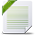# Power BI Exchange

View Only

## How to reference within a calculated column?#### Tom PaulsonJun 29, 2022 12:00 AM#### Marc SchroyenJun 29, 2022 03:30 PM• #### 1.  How to reference within a calculated column?

Posted Jun 29, 2022 12:00 AM
Edited by Tom Paulson Jun 29, 2022 12:01 AM
I have the following Data:The idea is to identify and forecast demand for receipts every month of each year. This would be column G. Obviously, we don't know what demand will be for month (row) 1. However, we do know what the number of average yearly receipts issued is and the avergae receipts issued for each month is so we can use those figures as a starting point by taking take the AvgYearlyReceiptsIssued (All Years) and multiply it by the AvgPercentageOfReceiptsIssuedThisMonth (All Years).

For each month that follows, demand is to be determined by subtracting the expected demand of the previous month and subtracting the number of receipts issued in that previous month and adding the result to our average monthly issued receipts baseline. This process repeats for every month until the end of the table.

The below is a breakdown of the expected results for column G:
Row      Calculation              Expected Result
1 D1*E1 339478
2 (D2*E2)+(G1-F2) 52319
3 (D3*E3)+(G2-F3) -105376
4 (D4*E4)+(G3-F4) -163264
5 (D5*E5)+(G4-F5) -221152
6 (D6*E6)+(G5-F6) -55425
7 (D7*E7)+(G6-F7) -246910

At issue, is the fact that the calculation requires column G to self-reference and iterate. My guess is that this requires using some sort of temporary table that exists inside of a loop but I have no idea how to go about this, let alone achieve the result.

Any ideas?

------------------------------
Tom Paulson
------------------------------

• #### 2.  RE: How to reference within a calculated column?

Posted Jun 29, 2022 03:30 PM
Hi Paul,

You can't and you don't need to self-reference.
Formula are calculated in a context, we need to define the proper context.
(D2*E2)+(G1-F2) can be transformed as:
(D2*E2)+(D1*E1)-F2)
(D3*E3)+(D2*E2)+(D1*E1)-F2-F3) and so on
==> iterator
This code should work
``````Result =
VAR CurrentDate = MAX( 'Table'[Start of Month] )
VAR PreviousRow = CALCULATE( MAX( 'Table'[Start of Month] ), 'Table'[Start of Month] < CurrentDate )
VAR PreviousReceiptsIssued =
CALCULATE(
SUM( 'Table'[Receipts issued] ),
REMOVEFILTERS( 'Table'[Start of Month] ),
'Table'[Start of Month] < CurrentDate
)
VAR G =
CALCULATE(
SUMX(
'Table',
'Table'[%AvgPercentageOfReceiptsissued This Month(All Years)]
* 'Table'[AvgYearlyReceiptsissued(All Years)]
),
REMOVEFILTERS( 'Table'[Start of Month] ),
'Table'[Start of Month] <= CurrentDate
)
RETURN
G - PreviousReceiptsIssued​``````

Here is the result:There is discrepancy with your expected result, but applying your formula in Excel gives the same result.
If I missed anything, let me know.
Checked by yourself in the pbix uploaded

------------------------------
Marc Schroyen
Liège Belgium
------------------------------

Attachment(s)Paulson.pbix   23 KB 1 version

• #### 3.  RE: How to reference within a calculated column?

Posted Jun 29, 2022 05:40 PM
Amazing! The discrepancy is caused by my rounding the E1 value in my calculations whereas your solution doesn't. Thank you!

------------------------------
Tom Paulson
------------------------------